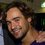# Euler-Fermat extended

An often useful theorem in number theory is Euler's theorem (also known as the Fermat–Euler theorem or Euler's totient theorem), which says that:

If $a$ and $n$ are coprime positive integers, then

$a^{\phi(n)}\equiv 1 (\text{mod }n)$

where $\phi(n)$ is Euler's totient function, counting how many of the integers $1,2,\ldots,n$ are coprime with $n$.

If, however, $a$ and $n$ are not coprime, we know for sure that the above does not hold. After all, if $a$ and $n$ share a common factor (greater than 1), any power of $a$ will also share this factor and will not equal 1 modulo $n$. However, if we formulate the equivalence as:

$a^{\phi(n)+1}\equiv a (\text{mod }n)$

(which, as it turns out, is equivalent to the original for $a,n$ coprime), there are certain cases where this relation still holds even if $a$ and $n$ do share a common factor. A sufficient (though not necessary) condition is for $n$ to be squarefree. Thus we obtain the following theorem:

Theorem. If $a,n$ are positive integers where $n$ is squarefree, then:

$a^{\phi(n)+1}\equiv a (\text{mod }n)$

where $\phi(n)$ is Euler's totient function.

Proof.

Let $n=p_1p_2\dots p_k$, where all the $p_i$ are distinct primes. We can write $a=bp_{c_1}p_{c_2}\dots p_{c_m}$, where the $c_i\in\{1,2,\ldots,k\}$ are all distinct and $b$ and $n$ are coprime. Modulo $p_{c_i}$ (for any $i=1,2,\ldots, m$) we have $a^{\phi(n)+1}\equiv 0 \equiv a (\text{mod }p_{c_1})$ since, of course, $p_{c_1}$ divides $a$. Analogously we know the same for all the other $p_{c_i}$. Furthermore, we have:

$a^{\phi(n)}\equiv 1 \left(\text{mod }\frac{n}{p_{c_1}\dots p_{c_m}}\right)$

since $a$ and $\frac{n}{p_{c_1}\dots p_{c_m}}$ are coprime, and $\phi\left(\frac{n}{p_{c_1}\dots p_{c_m}}\right)$ divides $\phi(n)$. Hence we obtain the following system:

\begin{aligned} a^{\phi(n)+1} &\equiv a (\text{mod }p_{c_1})\\ &\vdots\\ a^{\phi(n)+1} &\equiv a (\text{mod }p_{c_m})\\ a^{\phi(n)+1} &\equiv a \left(\text{mod }\frac{n}{p_{c_1}\dots p_{c_m}}\right). \end{aligned}

Since all the modulo classes on the right are coprime, we can use the Chinese remainder theorem to see that there exists a unique solution for this system, namely

$a^{\phi(n)+1} \equiv a \left(\text{mod }p_{c_1}\dots p_{c_m}\frac{n}{p_{c_1}\dots p_{c_m}}\right)$

or, in other words:

$a^{\phi(n)+1} \equiv a (\text{mod } n).\qquad\square$

This shows us, for example, that $a^5\equiv a (\text{mod }10)$ for any $a$, since $\phi(10)=4$ and $10$ is squarefree. Hence the last digit of any number is equal to the last digit of its fifth power, a very useful fact in for example the problem which inspired this note.Note by Tijmen Veltman
6 years, 2 months ago

This discussion board is a place to discuss our Daily Challenges and the math and science related to those challenges. Explanations are more than just a solution — they should explain the steps and thinking strategies that you used to obtain the solution. Comments should further the discussion of math and science.

When posting on Brilliant:

• Use the emojis to react to an explanation, whether you're congratulating a job well done , or just really confused .
• Ask specific questions about the challenge or the steps in somebody's explanation. Well-posed questions can add a lot to the discussion, but posting "I don't understand!" doesn't help anyone.
• Try to contribute something new to the discussion, whether it is an extension, generalization or other idea related to the challenge.

MarkdownAppears as
*italics* or _italics_ italics
**bold** or __bold__ bold
- bulleted- list
• bulleted
• list
1. numbered2. list
1. numbered
2. list
Note: you must add a full line of space before and after lists for them to show up correctly
paragraph 1paragraph 2

paragraph 1

paragraph 2

[example link](https://brilliant.org)example link
> This is a quote
This is a quote
    # I indented these lines
# 4 spaces, and now they show
# up as a code block.

print "hello world"
# I indented these lines
# 4 spaces, and now they show
# up as a code block.

print "hello world"
MathAppears as
Remember to wrap math in $$ ... $$ or $ ... $ to ensure proper formatting.
2 \times 3 $2 \times 3$
2^{34} $2^{34}$
a_{i-1} $a_{i-1}$
\frac{2}{3} $\frac{2}{3}$
\sqrt{2} $\sqrt{2}$
\sum_{i=1}^3 $\sum_{i=1}^3$
\sin \theta $\sin \theta$
\boxed{123} $\boxed{123}$